2021 NFL Defensive Rookie of the Year Odds
+300
3 to 1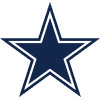Micah Parsons
17.1% implied probability

+700
7 to 1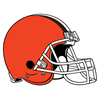Jeremiah Owusu-Koramoah
8.5% implied probability

+800
8 to 1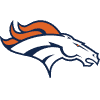Patrick Surtain
7.6% implied probability

+1000
10 to 1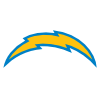Asante Samuel
6.2% implied probability

+1000
10 to 1Jamin Davis
6.2% implied probability

+1600
16 to 1Joe Tryon-Shoyinka
4.0% implied probability

+2500
25 to 1Osa Odighizuwa
2.6% implied probability

+2500
25 to 1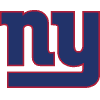Azeez Ojulari
2.6% implied probability

+3000
30 to 1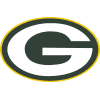Eric Stokes
2.2% implied probability

+4000
40 to 1Zaven Collins
1.7% implied probability

+4000
40 to 1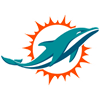Jevon Holland
1.7% implied probability

+4000
40 to 1Jaycee Horn
1.7% implied probability

+4000
40 to 1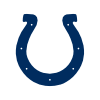Kwity Paye
1.7% implied probability

+4000
40 to 1Greg Newsome
1.7% implied probability

+4000
40 to 1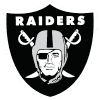Trevon Moehrig
1.7% implied probability

+5000
50 to 1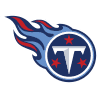Caleb Farley
1.3% implied probability

+5000
50 to 1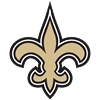Pete Werner
1.3% implied probability

+5000
50 to 1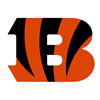Joseph Ossai
1.3% implied probability

+5000
50 to 1Justin Hilliard
1.3% implied probability

+5000
50 to 1Jabril Cox
1.3% implied probability

+5000
50 to 1Baron Browning
1.3% implied probability

+5000
50 to 1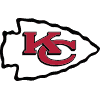Nick Bolton
1.3% implied probability

+6500
65 to 1Payton Turner
1.0% implied probability

+6500
65 to 1Kelvin Joseph
1.0% implied probability

+6500
65 to 1Jamar Johnson
1.0% implied probability

+6500
65 to 1Aaron Robinson
1.0% implied probability

+6500
65 to 1Andre Cisco
1.0% implied probability

+10000
100 to 1Daviyon Nixon
0.7% implied probability

+10000
100 to 1Tommy Togiai
0.7% implied probability

+10000
100 to 1Nate Hobbs
0.7% implied probability

+10000
100 to 1Ar'Darius Washington
0.7% implied probability

+10000
100 to 1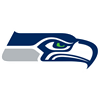Tre Brown
0.7% implied probability

+10000
100 to 1Hamsah Nasirildeen
0.7% implied probability

+10000
100 to 1Divine Deablo
0.7% implied probability

+10000
100 to 1Benjamin St-Juste
0.7% implied probability

+10000
100 to 1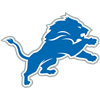Derrick Barnes
0.7% implied probability

+10000
100 to 1Elijah Molden
0.7% implied probability

+10000
100 to 1Tyson Campbell
0.7% implied probability

+10000
100 to 1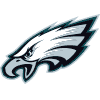Tay Gowan
0.7% implied probability

+10000
100 to 1Cameron Sample
0.7% implied probability

+10000
100 to 1Milton Williams
0.7% implied probability

+10000
100 to 1Rashad Weaver
0.7% implied probability

+10000
100 to 1Ifeatu Melifonwu
0.7% implied probability

+10000
100 to 1Carlos Basham
0.7% implied probability

+10000
100 to 1Dayo Odeyingbo
0.7% implied probability

+10000
100 to 1Marvin Wilson
0.7% implied probability

+10000
100 to 1Levi Onwuzurike
0.7% implied probability

+10000
100 to 1Thomas Graham
0.7% implied probability

+10000
100 to 1Tyler Shelvin
0.7% implied probability

+10000
100 to 1Ronnie Perkins
0.7% implied probability

+10000
100 to 1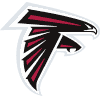Richie Grant
0.7% implied probability

+10000
100 to 1Christian Barmore
0.7% implied probability

+10000
100 to 1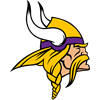Chazz Surratt
0.7% implied probability

+10000
100 to 1Bobby Brown
0.7% implied probability